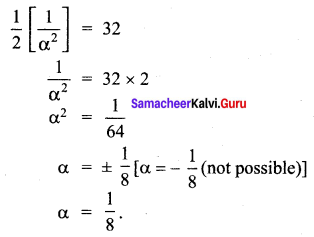## Tamilnadu Samacheer Kalvi 12th Maths Solutions Chapter 9 Applications of Integration Ex 9.7

Question 1.
Evaluate the following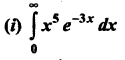Solution:
We know that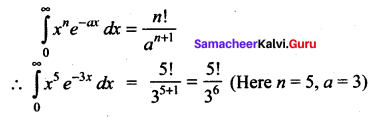(ii)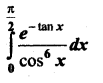Solution: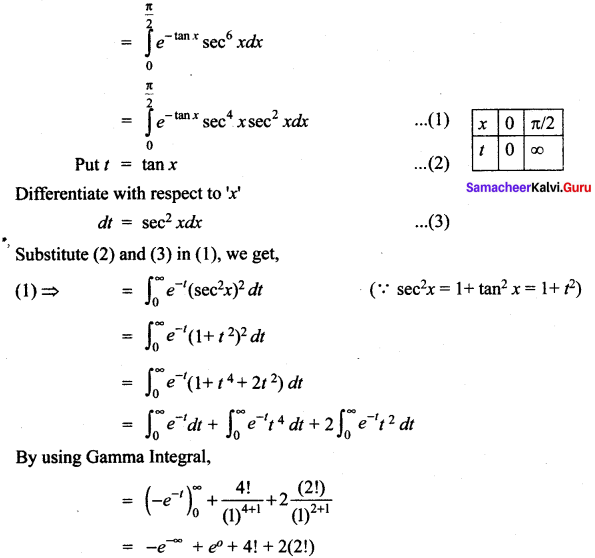= 0 + 1 + 24 + 4 = 29Question 2.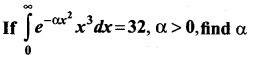Solution: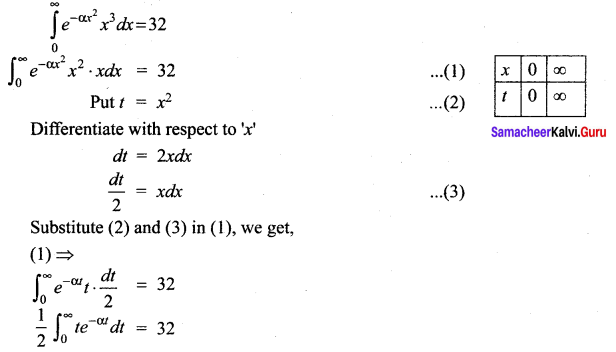By using Gamma integral (n = 1; a = α)